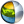Cg 3.1 Toolkit Documentation Cg / Standard Library / cos

## Name

cos - returns cosine of scalars and vectors.

## Synopsis

```float  cos(float a);
float1 cos(float1 a);
float2 cos(float2 a);
float3 cos(float3 a);
float4 cos(float4 a);

half   cos(half a);
half1  cos(half1 a);
half2  cos(half2 a);
half3  cos(half3 a);
half4  cos(half4 a);

fixed  cos(fixed a);
fixed1 cos(fixed1 a);
fixed2 cos(fixed2 a);
fixed3 cos(fixed3 a);
fixed4 cos(fixed4 a);
```

## Parameters

a
Vector or scalar of which to determine the cosine.

## Description

Returns the cosine of a in radians. The return value is in the range [-1,+1].

For vectors, the returned vector contains the cosine of each element of the input vector.

## Reference Implementation

cos is best implemented as a native cosine instruction, however cos for a float scalar could be implemented by an approximation like this.

```cos(float a)
{
/* C simulation gives a max absolute error of less than 1.8e-7 */
const float4 c0 = float4( 0.0,            0.5,
1.0,            0.0            );
const float4 c1 = float4( 0.25,          -9.0,
0.75,           0.159154943091 );
const float4 c2 = float4( 24.9808039603, -24.9808039603,
-60.1458091736,  60.1458091736  );
const float4 c3 = float4( 85.4537887573, -85.4537887573,
-64.9393539429,  64.9393539429  );
const float4 c4 = float4( 19.7392082214, -19.7392082214,
-1.0,            1.0            );

/* r0.x = cos(a) */
float3 r0, r1, r2;

r1.x  = c1.w * a;                       // normalize input
r1.y  = frac( r1.x );                   // and extract fraction
r2.x  = (float) ( r1.y < c1.x );        // range check: 0.0 to 0.25
r2.yz = (float2) ( r1.yy >= c1.yz );    // range check: 0.75 to 1.0
r2.y  = dot( r2, c4.zwz );              // range check: 0.25 to 0.75
r0    = c0.xyz - r1.yyy;                // range centering
r0    = r0 * r0;
r1    = c2.xyx * r0 + c2.zwz;           // start power series
r1    =     r1 * r0 + c3.xyx;
r1    =     r1 * r0 + c3.zwz;
r1    =     r1 * r0 + c4.xyx;
r1    =     r1 * r0 + c4.zwz;
r0.x  = dot( r1, -r2 );                 // range extract

return r0.x;
```

## Profile Support

cos is fully supported in all profiles unless otherwise specified.

cos is supported via an approximation (shown above) in the vs_1_1, vp20, and arbvp1 profiles.

cos is unsupported in the fp20, ps_1_1, ps_1_2, and ps_1_3 profiles.| Cg Toolkit | Download | Release Archive | Profiles | Reference | Books | Discussions |